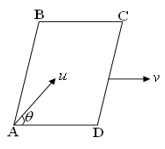Relative motion based problems
Question

# A smooth square platform ABCD is moving towards right with a uniform speed v.  At what angle $\mathrm{\theta }$  must a particle be projected from A with speed u so that it strikes the point B.Moderate
Solution

## When the relative velocity along horizontal will be zero, ie, $\mathrm{ucos\theta }=\mathrm{v} \mathrm{cos\theta }=\frac{\mathrm{v}}{\mathrm{u}}$

Get Instant Solutions